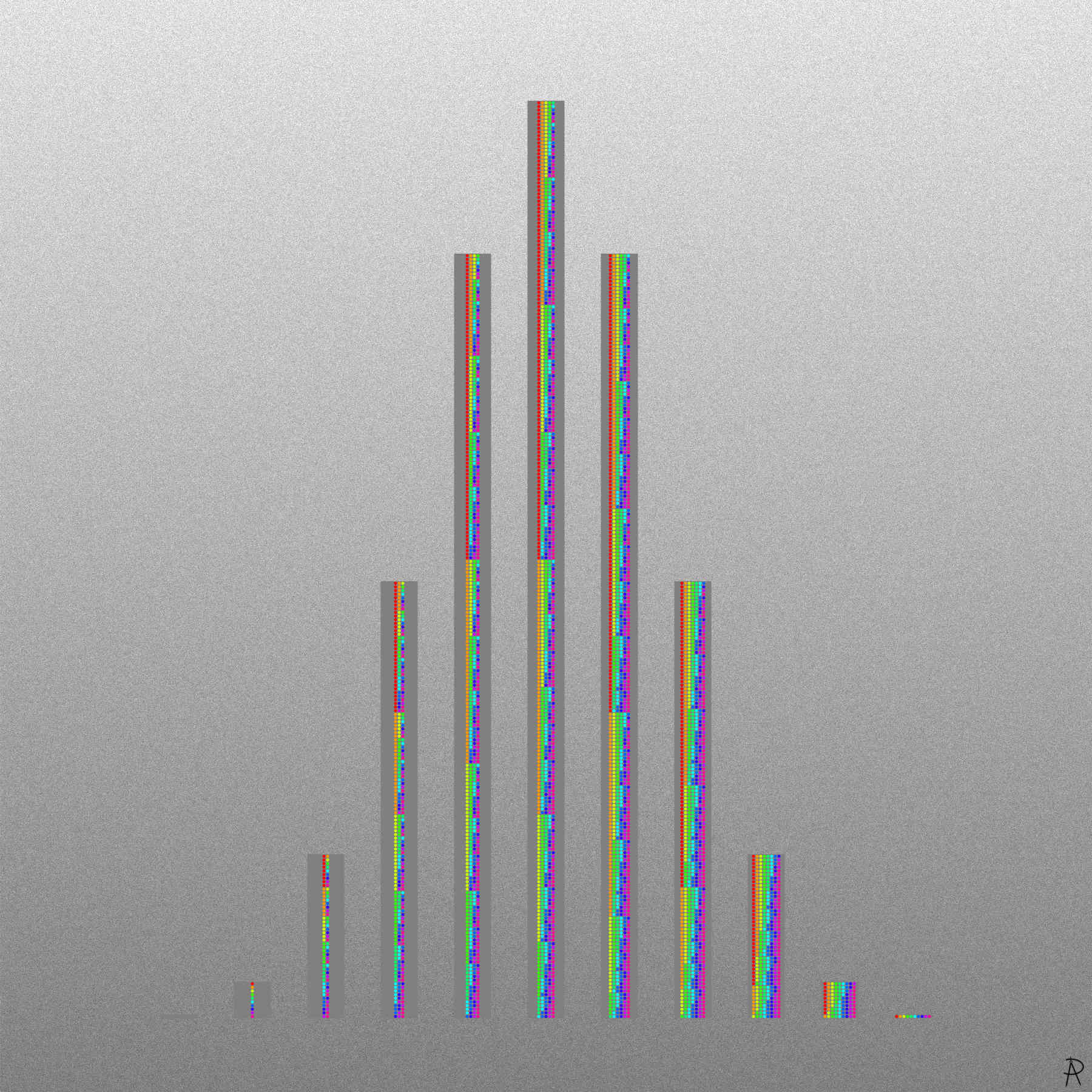Binomial Choices Digital Print 2021

All patterns of choosing items from a set of ten, from zero ( far left) to ten (far right). The patterns are grouped into the 11 possibilities for the number of choices in for a given size. The number in each group is a binomial coefficient related the number of ways to choose $$k$$ items from a set of 10 items, resulting in a binomial distribution.

### Background and Inspiration

This piece illustrates the way binomial coefficients relate to counting the number of ways of choosing $$k$$ items from a set of $$n$$ items.

The number of choosing $$k$$ items from a set of $$n$$ items is given by row $$n$$ of Pascal's triangle (see below). This artwork corresponds to $$n = 10$$. When $$k$$ is 0 or 10, there is only 1 possible pattern (no colors). When $$k$$ is 1 or 9, there are 10 possible patterns (all colors). For $$k$$ or $$10-k$$, equaling 2, 3, 4, and 5, the number of possible patterns are 45, 120, 210, and 252 respectively.

Each row in the column with $$k$$ colors can be thought of a set, with $$10 \choose k$$ subsets of the set with $$10$$ colors. Thus, there are $$2^{10} = 1024$$ total subsets that can be made from a set with $$10$$ colors.